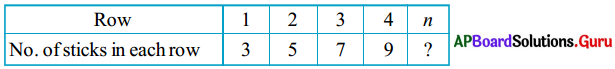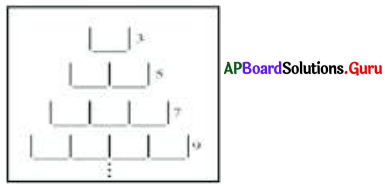# AP Board 7th Class Maths Solutions Chapter 9 Algebraic Expressions Review Exercise

SCERT AP 7th Class Maths Solutions Pdf Chapter 9 Algebraic Expressions Review Exercise Questions and Answers.

## AP State Syllabus 7th Class Maths Solutions 9th Lesson Algebraic Expressions Review Exercise

Question 1.
Identify constants and variables in the following terms:
0, – x, 3t, – 5, 5ab, – m, 700, – n, 2pqr, – 1, ab, 10, – 6z
Given, 0, – x, 3t, – 5, 5ab, – m, 700, – n, 2pqr, – 1, ab, 10, – 6z
Constants = 0, – 5, 700, – 1, 10
Variables = – x, 3t, 5ab, – m, – n, 2pqr, ab, – 6z.Question 2.
Observe the pattern the side and express the pattern in the form of an algebraic expression.In row = 1, 2(1) + 1 = 3
In row = 2, 2(2) + 1 = 5
In row = 3, 2(3) + 1 = 7
In row = 4, 2(4) + 1 = 9
In row = n, 2(n) + 1 = 2n + 1

Question 3.
Write the following statements as expressions
(i) x reduced by 5
Given x reduced by 5 = x – 5

(ii) 8 more than twice of k
Given 8 more than twice of k = 2k + 8

(iii) Half of y
Given Half of y = $$\frac{1}{2}$$ of y = $$\frac{y}{2}$$

(iv) One fourth of product of b and c
Given One fourth of product of b and c
= $$\frac{1}{4}$$ of b × c
= $$\frac{1}{4}$$ × bc = $$\frac{b c}{4}$$(v) One less than three times of p
Given One less than three times of p
= 3 times of p – 1
= 3 ∙ p – 1

Question 4.
Write the following expressions as statements :
(i) s + 3
Given s + 3 = 3 added to s.

(ii) 3p + 10
Given 3p + 10
= 10 more than thrice of p.

(iii) 5c – 8
Given 5c – 8 = 8 less than 5 times of c.

(iv) 10z
Given 10z = 10 times of z.

(v) $$\frac{b}{9}$$
Given $$\frac{b}{9}$$ = one nineth of b.

Question 5.
Write the following situations into algebraic expressions :
(i) Cost of one pen is double the cost of pencil.
Let the cost of pencil = ₹x
then the cost of pen = double the cost of pencil = 2 × ₹ x
∴ The cost of pen = ₹ 2x(ii) Age of John is 10 more than age of Yusuf.
Let the age of Yusuf = a years
then the age of John = (a + 10) years

(iii) Height of Siri is 15 cm less than height of Giri.